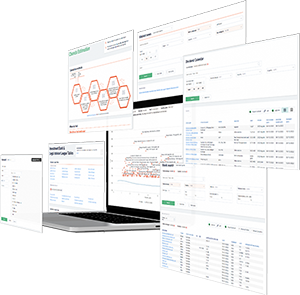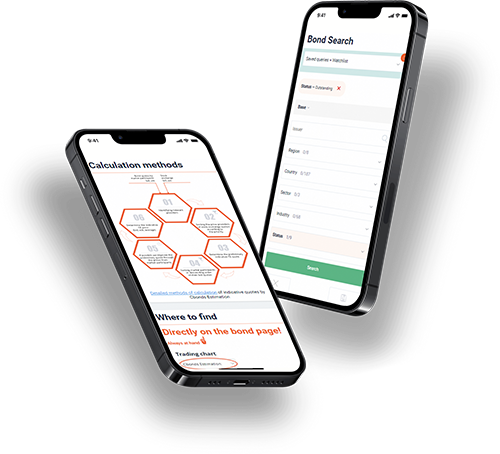Hint mode is switched on
Glossary

# Yield to Maturity

Category — Analytical Metrics

## What Is Meant by Yield to Maturity?

The yield to maturity (YTM) is calculated to determine the comprehensive expected return of a bond, considering its maturity date and other factors. The YTM represents the bond’s annual interest rate and is expressed as an annual rate. To calculate YTM, the bond’s annual coupon rate, maturity formula, and specific YTM formula are utilized.

In the process of calculating YTM, various elements come into play. These include the bond’s coupon rate, which determines the interest payments made to bondholders. The maturity calculation accounts for the bond’s maturity date, indicating when it reaches its full term. YTM is then calculated using a formula that incorporates these factors along with the bond’s current market value.

Bond investors commonly use YTM to evaluate fixed-income investments and compare different bonds. By estimating the annual rate of return through YTM, investors can assess the expected returns from their bond investments. It also allows them to compare bonds with varying coupon rates, maturities, and yields.

## Understanding Yield to Maturity

Yield to maturity, also known as book yield or redemption yield, shares similarities with current yield. Current yield divides the annual cash inflows from a bond by its market price to determine the potential one-year return from purchasing and holding the bond.

However, YTM goes beyond the current yield by incorporating the present value of a bond’s future coupon payments. By considering the time value of money, YTM provides a more comprehensive assessment of a bond’s return compared to a simple current yield calculation. Consequently, it is regarded as a more thorough approach to evaluating bond returns.

Analyzing the YTM of a discount bond without coupon payments serves as a useful starting point to grasp the intricacies associated with coupon bonds.

## Yield to Maturity vs. Coupon Rate

Investing in bonds presents a relatively low-risk opportunity to grow your funds. Unlike stocks, bond issuers guarantee the repayment of the bond’s full face value upon maturity. Nonetheless, investors must conduct thorough research before making any investment decisions, especially when considering bond purchases.

Two essential metrics accompany bonds: Yield to Maturity (YTM) and the coupon rate. YTM represents the anticipated overall return on a bond if it is held until maturity. On the other hand, the coupon rate, also known as the coupon yield, denotes the total income the bondholder receives from the bond for its duration. It signifies the annual interest payment calculated based on the bond’s face value.

While a bond’s YTM experiences fluctuations over time, the coupon rate remains fixed. When purchasing a bond at its face value, both the YTM and the coupon rate are equivalent. Opting for a bond at a discount implies a higher YTM, whereas acquiring a premium bond (above face value) results in a higher coupon rate.

## How is Yield to Maturity Calculated?

Yield to Maturity (YTM) represents the interest rate an investor would earn by reinvesting each coupon payment from the bond at a consistent interest rate until the bond reaches its maturity date. To determine the bond’s market price, the present value of all future cash flows must equal the bond’s current price.

Although an investor knows the bond’s current price, coupon payments, and maturity value, the discount rate cannot be directly calculated. However, a trial-and-error method can be employed by adjusting the YTM variable in the equation until the present value of the payment stream aligns with the bond’s price.

The formula to calculate YTM can be expressed as follows:

YTM = [(C + ((F - P) / n)) / ((F + P) / 2)] * (1 / ((T + 1) / 2)) - 1

Where:

• YTM = Yield to Maturity

• C = Periodic coupon payment

• F = Face value of the bond

• P = Current market price of the bond

• n = Number of coupon payments per year

• T = Number of years remaining until maturity

Solving this equation manually requires understanding the relationship between a bond’s price and its yield, as well as the different types of bond prices. Bonds can be priced at a discount, at par, or a premium. A bond priced at par indicates that its interest rate is equivalent to the coupon rate. Conversely, a premium bond, priced above par, possesses a coupon rate higher than the realized interest rate. On the other hand, a discount bond, priced below par, has a coupon rate lower than the realized interest rate.

When calculating YTM for a bond priced below par, an investor would input various annual interest rates higher than the coupon rate into the equation until finding a bond price close to the target bond’s price.

Yield-to-maturity calculations assume that all coupon payments are reinvested at the same rate as the bond’s current yield. It considers the bond’s current market price, par value, coupon interest rate, and remaining term to maturity. It is important to note that the YTM is a snapshot of the bond’s return, as coupon payments may not always be reinvested at the same interest rate. As interest rates rise, the YTM increases, while it decreases when interest rates decline.

Given the intricacies involved in determining yield to maturity, obtaining an exact YTM value can often be challenging. However, approximate YTM can be estimated using bond yield tables, financial calculators, or online YTM calculators.

## What Is an Example of Yield to Maturity?

Let’s consider an example where we calculate the yield of a bond. The bond has a maturity date when it reaches its full term, and its yield represents the annual interest rate it offers. To approximate the yield, we can use the bond’s coupon rate, which determines its interest payments. To calculate the yield to maturity (YTM), we employ a specific formula that considers the bond’s features and the time until maturity: YTM = [(C + ((F - P) / n)) / ((F + P) / 2)] * (1 / ((T + 1) / 2)) - 1.

Certainly! Let’s consider an example to calculate the yield to maturity (YTM) using the formula provided.

Suppose we have a bond with the following characteristics:

• Face value (F): \$1,000

• Coupon rate (C): 6% per annum

• Current market price (P): \$950

• Number of coupon payments per year (n): 2

• Years remaining until maturity (T): 5

Using the formula: YTM = [(C + ((F - P) / n)) / ((F + P) / 2)] * (1 / ((T + 1) / 2)) - 1

Let’s substitute the values into the formula:

YTM = [(0.06 + ((1000 - 950) / 2)) / ((1000 + 950) / 2)] * (1 / ((5 + 1) / 2)) - 1

Simplifying further:

YTM = [(0.06 + (50 / 2)) / (1975 / 2)] * (1 / (6 / 2)) - 1

YTM = [(0.06 + 25) / 987.5] * (1 / 3) - 1

YTM = (0.085 / 987.5) * (1 / 3) - 1

YTM = 0.00008611 * 0.3333 - 1

YTM = 0.0000287 - 1

YTM = -0.9999713

To convert the YTM to a percentage, we multiply it by 100:

YTM = -0.9999713 * 100

YTM = -99.99713%

Therefore, the yield to maturity (YTM) for this bond is approximately -99.99713%, indicating that the bond is likely trading at a discount compared to its face value.

This calculation is essential for fixed-income investments. A maturity calculator helps estimate the annual rate of return based on the bond’s maturity rate. Bond investors use these calculations to compare bonds and make informed investment decisions. The yield-to-maturity formula considers both the coupon rate and the price at which the bond is currently trading. As yields rise, the current value of the bond may fluctuate. To simplify these calculations, an online YTM calculator can be used. These calculations are crucial in personal finance and investment banking, particularly when dealing with debt securities with fixed prices and expected returns.

## What is Yield to Maturity used for?

Yield to maturity serves as a valuable tool for assessing the viability of a bond investment. An investor establishes a required yield, which represents the desired return on a bond that would make the investment worthwhile. Once the YTM of a prospective bond purchase has been determined, it can be compared against the required yield to evaluate the attractiveness of the bond as an investment.

Since YTM is expressed as an annual rate, regardless of the bond’s specific term to maturity, it enables the comparison of bonds with varying maturities and coupon rates. By employing YTM, the value of different bonds can be assessed using a consistent annual metric, facilitating meaningful comparisons across the bond market.

## YTM Variations

• Yield to call (YTC) takes into account the likelihood of the bond being called before its maturity. In this case, the issuer repurchases the bond, resulting in a shortened cash flow period. YTC is calculated assuming the bond will be called as soon as it becomes financially feasible and permissible.

• Yield to put (YTP) resembles YTC, but it applies to bonds where the bondholder has the option to sell the bond back to the issuer at a predetermined price based on the bond’s terms. YTP is calculated based on the assumption that the bond will be put back to the issuer as soon as it becomes financially feasible and permissible.

• Yield to worst (YTW) is employed when a bond incorporates multiple options. For instance, if an investor evaluates a bond with both call and put provisions, the YTW is calculated based on the option terms that yield the lowest possible return. It represents the scenario where the bond’s worst possible yield is realized.

## Limitations of Yield to Maturity

Yield-to-maturity (YTM) calculations typically do not incorporate the impact of taxes paid by an investor on the bond. YTM is referred to as the gross redemption yield when taxes are not factored in. Additionally, YTM calculations do not consider the costs associated with purchasing or selling the bond.

YTM also relies on certain assumptions about the future that are inherently uncertain. For instance, an investor may not have the opportunity to reinvest all coupon payments, the bond may not be held until maturity as initially anticipated, and the bond issuer may default on their payment obligations. These uncertainties introduce potential deviations from the calculated YTM.

## Find Any Data on Any Bond in Just One Click### Full data on over 500,000 bonds and stocks worldwide### Powerful bond screener### Ratings from the top 3 global ratings agencies, plus over 70 local ones### Over 300 pricing sources from the OTC market and world stock exchangesGet access
×

## — We have everything you need:full data on over 700 000 bonds, stocks & ETFs; powerful bond screener; over 350 pricing sources among stock exchanges & OTC market; ratings & financial reports; user-friendly interface; available anywhere via Website, Excel Add-in and Mobile app.

×

## WhyYou will have detailed descriptive & pricing data for 650K bonds, 76K stocks, 8K ETFsGet full access to the platform from any device & via Cbonds app